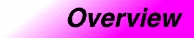# functorsCategory : functors Component type: overview

## Summary

A Function Object, or Functor (the two terms are synonymous) is simply any object that can be called as if it is a function. An ordinary function is a function object, and so is a function pointer; more generally, so is an object of a class that defines `operator()`.

## Description

The basic function object concepts are Generator, UnaryFunction, and BinaryFunction : these describe, respectively, objects that can be called as `f()`, `f(x)`, and `f(x,y)`. (This list could obviously be extended to ternary function and beyond, but, in practice, no STL algorithms require function objects of more than two arguments.) All other function object concepts defined by the STL are refinements of these three.

Function objects that return `bool` are an important special case. A UnaryFunction whose return type is `bool` is called a Predicate, and a BinaryFunction whose return type is `bool` is called a BinaryPredicate.

There is an important distinction, but a somewhat subtle one, between function objects and adaptable function objects.  In general, a function object has restrictions on the type of its argument. The type restrictions need not be simple, though : `operator()` may be overloaded, or may be a member template, or both. Similarly, there need be no way for a program to determine what those restrictions are. An adaptable function object, however, does specify what the argument and return types are, and provides nested `typedef`s so that those types can be named and used in programs. If a type `F0` is a model of AdaptableGenerator, then it must define `F0 result_type`. Similarly, if `F1` is a model of AdaptableUnaryFunction then it must define `F1 argument_type` and `F1::result_type`, and if `F2` is a model of AdaptableBinaryFunction then it must define `F2 first_argument_type`, `F2::second_argument_type`, and `F2::result_type`. The STL provides base classes `unary_function` and `binary_function` to simplify the definition of AdaptableUnaryFunction and AdaptableBinaryFunction. 

Adaptable function objects are important because they can be used by function object adaptors : function objects that transform or manipulate other function objects. The STL provides many different function object adaptors, including `unary_negate` (which returns the logical complement of the value returned by a particular AdaptablePredicate), and `unary_compose` and `binary_compose`, which perform composition of function object.

Finally, the STL includes many different predefined function objects, including arithmetic operations (`plus`, `minus`, `multiplies`, `divides`, `modulus`, and `negate`), comparisons (`equal_to`, `not_equal_to` `greater`, `less`, `greater_equal`, and `less_equal`), and logical operations (`logical_and`, `logical_or`, and `logical_not`). It is possible to perform very sophisticated operations without actually writing a new function object, simply by combining predefined function objects and function object adaptors.

## Examples

Fill a `Vector` with random numbers. In this example, the function object is simply a function pointer.

```    Vector<int> V(100);
generate(V.begin(), V.end(), rand);
```

Sort a `Vector` of `double` by magnitude, i.e. ignoring the elements' signs. In this example, the function object is an object of a user-defined class.

```    struct less_mag  : public binary_function<double, double, bool> {
bool operator()(double x, double y) { return fabs(x) < fabs(y); }
};

Vector<double> V;
...
sort(V.begin(), V.end(), less_mag());
```

Find the sum of elements in a `Vector`. In this example, the function object is of a user-defined class that has local state.

```    struct adder  : public unary_function<double, void>
{
double sum;
void operator()(double x) { sum += x; }
};

Vector<double> V;
...
cout << "The sum is " << result.sum << endl;
```

Remove all elements from a `List` that are greater than 100 and less than 1000.

```    List<int> L;
...
List<int> ::iterator new_end =
remove_if(L.begin(), L.end(),
compose2(logical_and<bool>(),
bind2nd(greater<int>(), 100),
bind2nd(less<int>(), 1000)));
L.erase(new_end, L.end());
```

## Types

• `plus`
• `minus`
• `multiplies` (formerly called `times`)
• `divides`
• `modulus`,
• `negate`
• `equal_to`
• `not_equal_to`
• `greater`
• `less`
• `greater_equal`
• `less_equal`,
• `logical_and`
• `logical_or`
• `logical_not`
• `subtractive_rng`
• `identity`
• `project1st`
• `project2nd`
• `select1st`
• `select2nd`
• `unary_function`
• `binary_function`
• `unary_compose`
• `binary_compose`
• `unary_negate`
• `binary_negate`
• `binder1st`
• `binder2nd`
• `pointer_to_unary_function`
• `pointer_to_binary_function`

## Functions

• `compose1`
• `compose2`
• `not1`
• `not2`
• `bind1st`
• `bind2nd`
• `ptr_fun`